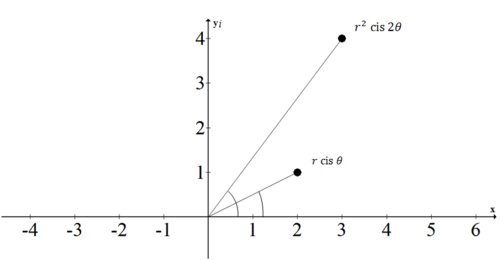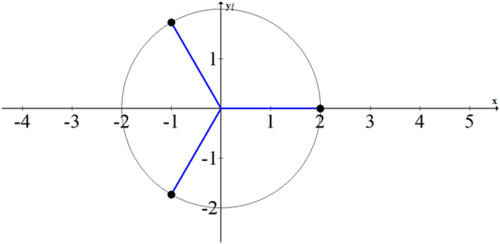# 11.4 De Moivre's Theorem and nth Roots

$$\newcommand{\vecs}{\overset { \rightharpoonup} {\mathbf{#1}} }$$ $$\newcommand{\vecd}{\overset{-\!-\!\rightharpoonup}{\vphantom{a}\smash {#1}}}$$$$\newcommand{\id}{\mathrm{id}}$$ $$\newcommand{\Span}{\mathrm{span}}$$ $$\newcommand{\kernel}{\mathrm{null}\,}$$ $$\newcommand{\range}{\mathrm{range}\,}$$ $$\newcommand{\RealPart}{\mathrm{Re}}$$ $$\newcommand{\ImaginaryPart}{\mathrm{Im}}$$ $$\newcommand{\Argument}{\mathrm{Arg}}$$ $$\newcommand{\norm}{\| #1 \|}$$ $$\newcommand{\inner}{\langle #1, #2 \rangle}$$ $$\newcommand{\Span}{\mathrm{span}}$$ $$\newcommand{\id}{\mathrm{id}}$$ $$\newcommand{\Span}{\mathrm{span}}$$ $$\newcommand{\kernel}{\mathrm{null}\,}$$ $$\newcommand{\range}{\mathrm{range}\,}$$ $$\newcommand{\RealPart}{\mathrm{Re}}$$ $$\newcommand{\ImaginaryPart}{\mathrm{Im}}$$ $$\newcommand{\Argument}{\mathrm{Arg}}$$ $$\newcommand{\norm}{\| #1 \|}$$ $$\newcommand{\inner}{\langle #1, #2 \rangle}$$ $$\newcommand{\Span}{\mathrm{span}}$$$$\newcommand{\AA}{\unicode[.8,0]{x212B}}$$

You know how to multiply two complex numbers together and you’ve seen the advantages of using trigonometric polar form, especially when multiplying more than two complex numbers at the same time. Because raising a number to a whole number power is repeated multiplication, you also know how to raise a complex number to a whole number power. What is a geometric interpretation of squaring a complex number?

## De Moivre's Theorem and nth Roots

Recall that if $$z_{1}=r_{1} \cdot \operatorname{cis} \theta_{1}$$ and $$z_{2}=r_{2} \cdot \operatorname{cis} \theta_{2}$$ with $$r_{2} \neq 0$$, then $$z_{1} \cdot z_{2}=r_{1} \cdot r_{2} \cdot \operatorname{cis}\left(\theta_{1}+\theta_{2}\right)$$

If $$z_{1}=z_{2}=z=r \operatorname{cis} \theta$$ then you can determine $$z^{2}$$ and $$z^{3}$$ :

$$z^{2}=r \cdot r \cdot \operatorname{cis}(\theta+\theta)=r^{2} \operatorname{cis}(2 \cdot \theta)$$

$$z^{3}=r^{3} \operatorname{cis}(3 \cdot \theta)$$

De Moivre's Theorem simply generalizes this pattern to the power of any positive integer.

$$z^{n}=r^{n} \cdot \operatorname{cis}(n \cdot \theta)$$

In addition to raising a complex number to a power, you can also take square roots, cube roots and $$n^{\text {th }}$$ roots of complex numbers. Suppose you have complex number $$z=r$$ cis $$\theta$$ and you want to take the $$n^{t h}$$ root of $$z$$. In other words, you want to find a number $$v=s \cdot \operatorname{cis} \beta$$ such that $$v^{n}=z$$. Do some substitution and manipulation:

\begin{aligned} v^{n} &=z\\(s \cdot \operatorname{cis} \beta)^{n} &=r \cdot \operatorname{cis} \theta \\ s^{n} \cdot \operatorname{cis}(n \cdot \beta) &=r \cdot \operatorname{cis} \theta \end{aligned}

You can see at this point that to find $$s$$ you need to take the $$n^{\text {th }}$$ root of $$r$$. The trickier part is to find the angles, because $$n \cdot \beta$$ could be any angle coterminal with $$\theta$$. This means that there are $$n$$ different $$n^{\text {th }}$$ roots of $$z$$.

$$n \cdot \beta=\theta+2 \pi k$$

$$\beta=\frac{\theta+2 \pi k}{n}$$

The number $$k$$ can be all of the counting numbers including zeros up to $$n-1$$. So if you are taking the $$4^{\text {th }}$$ root, then $$k=0,1,2,3$$.

Thus the $$n^{t h}$$ root of a complex number requires $$n$$ different calculations, one for each root:

$$v=\sqrt[n]{r} \cdot \operatorname{cis}\left(\frac{\theta+2 \pi k}{n}\right)$$ for $$\{k \in I \mid 0 \leq k \leq n-1\}$$

To apply this formula, find the cube root of the number 8 . Most students know that $$2^{3}=8$$ and so know that 2 is the cube root of 8 . However, they don't realize that there are two other cube roots that they are missing. Remember to write out $$k=0,1,2$$ and use the unit circle whenever possible to help you to find all three cube roots.

\begin{aligned} 8 &=8 \text { cis } 0=(s \cdot \operatorname{cis} \beta)^{3} \\ z_{1} &=2 \cdot \operatorname{cis}\left(\frac{0+2 \pi \cdot 0}{3}\right)=2 \text { cis } 0=2(\cos 0+i \cdot \sin 0)=2(1+0)=2 \\ z_{2} &=2 \cdot \operatorname{cis}\left(\frac{0+2 \pi \cdot 1}{3}\right)=2 \operatorname{cis}\left(\frac{2 \pi}{3}\right) \\ &=2\left(\cos \left(\frac{2 \pi}{3}\right)+i \cdot \sin \left(\frac{2 \pi}{3}\right)\right)=2\left(-\frac{1}{2}+\frac{\sqrt{3}}{2} i\right)=-1+i \sqrt{3} \\ z_{3} &=2 \cdot \operatorname{cis}\left(\frac{0+2 \pi \cdot 2}{3}\right)=2 \operatorname{cis}\left(\frac{4 \pi}{3}\right) \\ &=2\left(\cos \left(\frac{4 \pi}{3}\right)+i \cdot \sin \left(\frac{4 \pi}{3}\right)\right)=2\left(-\frac{1}{2}-\frac{\sqrt{3}}{2} i\right)=-1-i \sqrt{3} \end{aligned}

The cube roots of 8 are $$2,-1+i \sqrt{3},-1-i \sqrt{3}$$.

To check, that they are the cube roots, cube them all simplify.

$$z_{1}^{3}=2^{3}=8$$

$$z_{2}^{3}=(-1+i \sqrt{3})^{3}$$

$$\quad=(-1+i \sqrt{3}) \cdot(-1+i \sqrt{3}) \cdot(-1+i \sqrt{3})$$

$$\quad=(1-2 i \sqrt{3}-3) \cdot(-1+i \sqrt{3})$$

$$\quad=(-2-2 i \sqrt{3}) \cdot(-1+i \sqrt{3})$$

$$\quad=2-2 i \sqrt{3}+2 i \sqrt{3}+6$$

$$\quad=8$$

Note how many steps and opportunities there are for making a mistake when multiplying

multiple terms in rectangular form. When you check $$z_{3},$$ use trigonometric polar form.

\begin{aligned} z_{3}^{3} &=2^{3} \operatorname{cis}\left(3 \cdot \frac{4 \pi}{3}\right) \\ &=8(\cos 4 \pi+i \cdot \sin 4 \pi) \\ &=8(1+0) \\ &=8 \end{aligned}

## Examples

##### Example 1

Earlier, you were asked what a geometric interpretation of squaring a complex number is. Squaring a complex number produces a new complex number. The angle gets doubled and the magnitude gets squared, so geometrically you see a rotation.##### Example 2

Plot the roots of 8 graphically and discuss any patterns you notice.The three points are equally spaced around a circle of radius 2. Only one of the points, $$2+0 i$$, is made up of only real numbers. The other two points have both a real and an imaginary component which is why they are off of the $$x$$ axis.

As you become more comfortable with roots, you can just determine the number of points that need to be evenly spaced around a certain radius circle and find the first point. The rest is just logic.

##### Example 3

What are the fourth roots of 16 cis $$48^{\circ} ?$$

There will be 4 points, each $$90^{\circ}$$ apart with the first point at 2 cis $$\left(12^{\circ}\right)$$.

$$2 \operatorname{cis}\left(12^{\circ}\right), 2 \operatorname{cis}\left(102^{\circ}\right), 2 \operatorname{cis}\left(192^{\circ}\right), 2 \operatorname{cis}\left(282^{\circ}\right)$$

##### Example 4

Solve for $$z$$ by finding the nth root of the complex number.

$$z^{3}=64-64 \sqrt{3} i$$

First write the complex number in cis form. Remember to identify $$k=0,1,2$$. This means the roots will appear every $$\frac{360^{\circ}}{3}=120^{\circ}$$.

$$z^{3}=64-64 \sqrt{3} i=128 \cdot \operatorname{cis} 300^{\circ}$$

$$z_{1}=128^{\frac{1}{3}} \cdot \operatorname{cis}\left(\frac{300}{3} \circ\right)=128^{\frac{1}{3}} \cdot \operatorname{cis}\left(100^{\circ}\right)$$

$$z_{2}=128^{\frac{1}{3}} \cdot \operatorname{cis}\left(220^{\circ}\right)$$

$$z_{3}=128^{\frac{1}{3}} \cdot \operatorname{cis}\left(340^{\circ}\right)$$

##### Example 5

Use De Moivre's Theorem to evaluate the following power.

$$(\sqrt{2}-\sqrt{2} i)^{6}$$

First write the number in trigonometric polar form, then apply De Moivre's Theorem and simplify.

\begin{aligned}(\sqrt{2}-\sqrt{2} i)^{6} &=\left(2 \operatorname{cis} 315^{\circ}\right)^{6} \\ &=2^{6} \cdot \operatorname{cis}\left(6 \cdot 315^{\circ}\right) \\ &=64 \cdot \operatorname{cis}\left(1890^{\circ}\right) \\ &=64 \cdot \operatorname{cis}\left(1890^{\circ}\right) \\ &=64 \cdot \operatorname{cis}\left(90^{\circ}\right) \\ &=64\left(\cos 90^{\circ}+i \cdot \sin 90^{\circ}\right) \\ &=64(0+i) \\ &=64 i \end{aligned}

##### Review

Use De Moivre's Theorem to evaluate each expression. Write your answers in rectangular form.

1. $$(1+i)^{5}$$

2. $$(1-\sqrt{3} i)^{3}$$

3. $$(1+2 i)^{6}$$

4. $$(\sqrt{3}-i)^{5}$$

5. $$\left(\frac{1}{2}+\frac{i \sqrt{3}}{2}\right)^{4}$$

6. Find the cube roots of $$3+4 i$$.

7. Find the $$5^{\text {th }}$$ roots of $$32 i$$.

8. Find the $$5^{\text {th }}$$ roots of $$1+\sqrt{5} i$$.

9. Find the $$6^{\text {th }}$$ roots of -64 and plot them on the complex plane.

10. Use your answers to $$\# 9$$ to help you solve $$x^{6}+64=0$$.

For each equation: a) state the number of roots, b) calculate the roots, and c) represent the roots graphically.

11. $$x^{3}=1$$

12. $$x^{8}=1$$

13. $$x^{12}=1$$

14. $$x^{4}=16$$

15. $$x^{3}=27$$

This page titled 11.4 De Moivre's Theorem and nth Roots is shared under a CK-12 license and was authored, remixed, and/or curated by CK-12 Foundation via source content that was edited to the style and standards of the LibreTexts platform; a detailed edit history is available upon request.